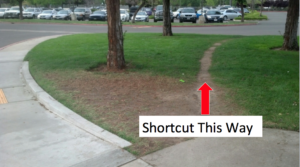## Special Cases – Squares

### Learning Outcomes

• Factor special productsSome people find it helpful to know when they can take a shortcut to avoid doing extra work. There are some polynomials that will always factor a certain way, and for those, we offer a shortcut. Most people find it helpful to memorize the factored form of a perfect square trinomial or a difference of squares. The most important skill you will use in this section will be recognizing when you can use the shortcuts.

## Factoring a Perfect Square Trinomial

A perfect square trinomial is a trinomial that can be written as the square of a binomial. Recall that when a binomial is squared, the result is the square of the first term added to twice the product of the two terms and the square of the last term.

$\begin{array}{ccc}\hfill {a}^{2}+2ab+{b}^{2}& =& {\left(a+b\right)}^{2}\hfill \\ & \text{and}& \\ \hfill {a}^{2}-2ab+{b}^{2}& =& {\left(a-b\right)}^{2}\hfill \end{array}$
We can use these equations to factor any perfect square trinomial.

### A General Note: Perfect Square Trinomials

A perfect square trinomial can be written as the square of a binomial:

${a}^{2}+2ab+{b}^{2}={\left(a+b\right)}^{2}$
${a}^{2}-2ab+{b}^{2}={\left(a-b\right)}^{2}$

In the following example, we will show you how to define a and b so you can use the shortcut.

### Example

Factor $25{x}^{2}+20x+4$.

In the next example, we will show that we can use $1 = 1^2$ to factor a polynomial with a term equal to $1$.

### Example

Factor $49{x}^{2}-14x+1$.

In the following video, we provide another short description of what a perfect square trinomial is and show how to factor them using a formula.

We can summarize our process in the following way:

### How To: Given a perfect square trinomial, factor it into the square of a binomial

1. Confirm that the first and last term are perfect squares.
2. Confirm that the middle term is twice the product of $ab$.
3. Write the factored form as ${\left(a+b\right)}^{2}$ or ${\left(a-b\right)}^{2}$.

## Factoring a Difference of Squares

A difference of squares is a perfect square subtracted from a perfect square. Recall that a difference of squares can be rewritten as factors containing the same terms but opposite signs because the middle terms cancel each other out when the two factors are multiplied.

${a}^{2}-{b}^{2}=\left(a+b\right)\left(a-b\right)$

We can use this equation to factor any differences of squares.

### A General Note: Differences of Squares

A difference of squares can be rewritten as two factors containing the same terms but opposite signs.

${a}^{2}-{b}^{2}=\left(a+b\right)\left(a-b\right)$

### Example

Factor $9{x}^{2}-25$.

The most helpful thing for recognizing a difference of squares that can be factored with the shortcut is knowing which numbers are perfect squares, as you will see in the next example.

### Example

Factor $81{y}^{2}-144$.

In the following video, we show another example of how to use the formula for factoring a difference of squares.

We can summarize the process for factoring a difference of squares with the shortcut this way:

### How To: Given a difference of squares, factor it into binomials

1. Confirm that the first and last term are perfect squares.
2. Write the factored form as $\left(a+b\right)\left(a-b\right)$.

Is there a formula to factor the sum of squares, $a^2+b^2$, into a product of two binomials?